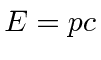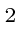## Sample Test Problems

1. What is the deBroglie wavelength of an electron with 13.6 eV of kinetic energy? What is the deBroglie wavelength of an electron with 10 MeV of kinetic energy?
13.6 eV is much less thanso this is non-relativistic.10 MeV is much bigger thanfor an electron so it is super-relativistic and we can use.2. What is the DeBroglie wavelength for each of the following particles? The energies given are the kinetic energies.
a)
a 10 eV electron
b)
a 1 MeV electron
c)
a 10 MeV proton
3. A 2 slit electron diffraction experiment is set up as (not) shown below. The observed electron intensity distribution is plotted in the figure. Now an intense light source is introduced near the two slits. With this light, it can be "seen" which slit each electron goes through. Qualitatively plot the new electron intensity distribution from each slit and from the 2 slits combined. What is the condition on the wavelength of the light for this effect to occur?
4. What is the DeBroglie wavelength for each of the following particles? The energies given are the kinetic energies.
a)
a 1 eV electron
b)
aMeV proton
5. What K.E. must a Hydrogen atom have so that its DeBroglie wavelength is smaller than the size of the atom? (Factors of 2 are not important.)
6. Calculate the DeBroglie wavelength for (a) a proton with 10 MeV kinetic energy, (b) An electron with 10 MeV kinetic energy, and (c) a 1 gram lead ball moving with a velocity of 10 cm/sec (one erg is one gram cm/sec). Be sure to take account of relativity where needed.

Jim Branson 2013-04-22